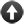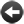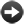§15.6. Powers and logarithms

If the last section provided a basic office calculator, this section and the next provide the more exotic rows of buttons found on a scientific calculator. All of these are done using real number arithmetic. To start with some dull ones, here are two ways to round off numbers:

ceiling of (real number) ... real number

Produces the smallest integer value greater than or equal to the one given. Examples:

ceiling of pi = 4.0
ceiling of -16.315 = -16.0

(Note that the result is still a real number; it simply has no fractional part any more.)

floor of (real number) ... real number

Produces the largest integer value less than or equal to the one given. Examples:

floor of pi = 3.0
floor of -16.315 = -17.0

(Note that the result is still a real number; it simply has no fractional part any more.)

Two more easy functions:

absolute value of (real number) ... real number

Removes the sign from a value, leaving positive numbers alone but making negative ones positive. Examples:

absolute value of 62.1 = 62.1
absolute value of 0 = 0.0
absolute value of -62.1 = 62.1
absolute value of minus infinity = plus infinity

reciprocal of (real number) ... real number

Calculates 1/x, that is, divides up 1 into this many pieces. Examples:

reciprocal of -2 = -0.5
reciprocal of 0.1 = 10.0
reciprocal of 7 = 0.14286
reciprocal of plus infinity = 0.0

Now for taking powers. In general we have:

(real number) to the power (real number) ... real number

Computes x to the power y. Examples:

2 to the power 4 = 16.0
100 to the power 0.5 = 10.0
7 to the power -1 = 0.14286
pi to the power 0 = 1.0

In the words of the Glulx specification document (section 2.12), "the special cases are breathtaking": if you need to know exactly what, say, "minus infinity to the power Y" will do for different cases of Y, refer to the details of the "pow" opcode.

To compute square roots, it's more efficient to use "real square root of X" function than "X to the power 0.5", though both work. To obtain the Nth root of X, we might use:

X to the power (reciprocal of N)

being careful to use "reciprocal of N" rather than "1 divided by N" to make sure we're using real and not integer arithmetic.

Similarly, the following is more efficient than "e to the power ...", but equivalent to it:

exponential of (real number) ... real number

Computes e to the given power, where e is the base of natural logarithms. Examples:

exponential of 0 = 1.0
exponential of 1 = e = 2.7182818
exponential of -10 = 4.53999 x 10^-5
exponential of 10 = 22026.46484
exponential of logarithm of 7.12 = 7.12

The reverse of taking powers is taking logarithms.

logarithm to base (number) of (real number) ... real number

Finds what power the base would have to be raised to in order to get this value. Examples:

logarithm to base 10 of 1000000 = 6.0
logarithm to base 10 of 350 = 2.54407
logarithm to base 2 of 256 = 8.0

Logarithms of zero or negative numbers are nonexistent. Note that "logarithm to base 10 of ..." is what most calculators call simply "log", but Inform doesn't: it uses "log" for natural logarithms.

natural/-- logarithm of (real number) ... real number

Finds what power e would have to be raised to in order to get this value. Examples:

logarithm of e = 1.0
logarithm of 1 = 0.0
logarithm of 1000 = 6.90776
logarithm of exponential of 7.12 = 7.12

Logarithms of zero or negative numbers are nonexistent. This is the function which most calculators label as "ln", for "log natural", but in mathematical and scientific papers it's more often written "log", and Inform follows that convention.Start of Chapter 15: Numbers and EquationsBack to §15.5. ArithmeticOnward to §15.7. Trigonometry